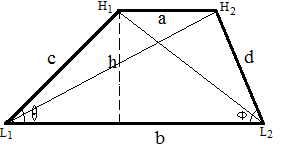## Trapezoid Calculator with Angles

 Parallel Side a: Parallel Side b: Angle (θ): radian  ̊ degree  π  π/3 Angle (Φ) radian  ̊ degree  π  π/3 Altitude (h): Area: Perimeter: Side c: Side d: Diagonal L1H2: Diagonal H1L2:

In Euclidean geometry, A trapezoid has two parallel sides are called the bases of the trapezoid. The other two sides are calld the lateral sides.

### Trapezoid Formulas1. Area = h(a+b)/2

2. Perimeter = a + b + c + d

3. Diagonal L1H2 = sqrt(h2+(b-sqrt(c2-h2))2)

4. Diagonal H1L2 = sqrt(h2+(b-sqrt(d2-h2))2)

For example, When Parallel Side a =3, b = 4, Angle (θ) = 0.9273, Angle (Φ) = 0.6082, altitude(h) = 4, Then the area= 14, perimeter = 19, Side c = 5, side d = 7, Diagonal L1H2 = 4.1231, Diagonal H1L2 = 4.3641.

Thinkcalculator.com provides you helpful and handy calculator resources.